ALL

Page: (Previous)   1  2  3  4  5  6  (Next)
ALL

### M

#### MAT-HS.G-SRT.03

 MAT-HS Targeted Standards(G) Concept: Geometry(SRT) Domain: Similarity, Right Triangle, and TrigonometryCluster: Understand similarity in terms of similarity transformations MAT-HS.G-SRT.03 Use the properties of similarity transformations to establish the AA criterion for two triangles to be similar.

## Student Learning Targets:

• I can
• I can

• I can
• I can

### Skills (Performance) Targets

• I can use the properties of similarity transformations to prove triangles are similar by AA criterion.

• I can
• I can

## Proficiency Scale

 Score Description Sample Activity 4.0 In addition to Score 3.0, the student demonstrates in-depth inferences and applications regarding more complex material that go beyond end of instruction expectations. - 3.5 In addition to Score 3.0 performance, the student demonstrates in-depth inferences and applications regarding the more complex content with partial success. 3.0 “The Standard.” The student demonstrates no major errors or omissions regarding any of the information and processes that were end of instruction expectations. - 2.5 The student demonstrates no major errors or omissions regarding the simpler details and processes (Score 2.0 content) and partial knowledge of the more complex ideas and processes (Score 3.0 content). 2.0 The student demonstrates no major errors or omissions regarding the simpler details and processes but exhibits major errors or omissions regarding the more complex ideas and processes (Score 3.0 content). - 1.5 The student demonstrates partial knowledge of the simpler details and processes (Score 2.0 content) but exhibits major errors or omissions regarding the more complex ideas and procedures (Score 3.0 content). 1.0 With help, the student demonstrates a partial understanding of some of the simpler details and processes (Score 2.0 content) and some of the more complex ideas and processes (Score 3.0 content). - 0.5 With help, the student demonstrates a partial understanding of some of the simpler details and processes (Score 2.0 content) but not the more complex ideas and processes (Score 3.0 content). 0.0 Even with help, the student demonstrates no understanding or skill. -

## Resources

 Web Vocab

#### MAT-HS.G-SRT.04

 MAT-HS Targeted Standards(G) Concept: Geometry(SRT) Domain: Similarity, Right Triangle, and TrigonometryCluster: Prove theorems involving similarity MAT-HS.G-SRT.04 Prove theorems about triangles. Theorems include: a line parallel to one side of a triangle divides the other two proportionally, and conversely; the Pythagorean Theorem proved using triangle similarity.

## Student Learning Targets:

• I can
• I can

• I can
• I can

### Skills (Performance) Targets

• I can use AAA, SAS, SSS similarity theorems to prove triangles are similar.
• I can use triangle similarity to prove other theorems about triangles.
• I can prove a line parallel to one side of a triangle divides the other two proportionally, and it’s converse.
• I can prove the Pythagorean Theorem using triangle similarity.

• I can
• I can

## Proficiency Scale

 Score Description Sample Activity 4.0 In addition to Score 3.0, the student demonstrates in-depth inferences and applications regarding more complex material that go beyond end of instruction expectations. - 3.5 In addition to Score 3.0 performance, the student demonstrates in-depth inferences and applications regarding the more complex content with partial success. 3.0 “The Standard.” The student demonstrates no major errors or omissions regarding any of the information and processes that were end of instruction expectations. - 2.5 The student demonstrates no major errors or omissions regarding the simpler details and processes (Score 2.0 content) and partial knowledge of the more complex ideas and processes (Score 3.0 content). 2.0 The student demonstrates no major errors or omissions regarding the simpler details and processes but exhibits major errors or omissions regarding the more complex ideas and processes (Score 3.0 content). - 1.5 The student demonstrates partial knowledge of the simpler details and processes (Score 2.0 content) but exhibits major errors or omissions regarding the more complex ideas and procedures (Score 3.0 content). 1.0 With help, the student demonstrates a partial understanding of some of the simpler details and processes (Score 2.0 content) and some of the more complex ideas and processes (Score 3.0 content). - 0.5 With help, the student demonstrates a partial understanding of some of the simpler details and processes (Score 2.0 content) but not the more complex ideas and processes (Score 3.0 content). 0.0 Even with help, the student demonstrates no understanding or skill. -

## Resources

 Web Vocab

#### MAT-HS.G-SRT.05

 MAT-HS Targeted Standards(G) Concept: Geometry(SRT) Domain: Similarity, Right Triangle, and TrigonometryCluster: Prove theorems involving similarity MAT-HS.G-SRT.05 Use congruence and similarity criteria for triangles to solve problems and to prove relationships in geometric figures.

## Student Learning Targets:

• I can
• I can

### Reasoning Targets

• I can determine congruence and similarity among geometric objects.
• I can use similarity or congruence to solve for a missing side/angle.

### Skills (Performance) Targets

• I can use similarity and congruence criteria to solve real world problems.
• I can use congruence and similarity to prove relationships involving geometric figures.

• I can
• I can

## Proficiency Scale

 Score Description Sample Activity 4.0 In addition to Score 3.0, the student demonstrates in-depth inferences and applications regarding more complex material that go beyond end of instruction expectations. - 3.5 In addition to Score 3.0 performance, the student demonstrates in-depth inferences and applications regarding the more complex content with partial success. 3.0 “The Standard.” The student demonstrates no major errors or omissions regarding any of the information and processes that were end of instruction expectations. - 2.5 The student demonstrates no major errors or omissions regarding the simpler details and processes (Score 2.0 content) and partial knowledge of the more complex ideas and processes (Score 3.0 content). 2.0 The student demonstrates no major errors or omissions regarding the simpler details and processes but exhibits major errors or omissions regarding the more complex ideas and processes (Score 3.0 content). - 1.5 The student demonstrates partial knowledge of the simpler details and processes (Score 2.0 content) but exhibits major errors or omissions regarding the more complex ideas and procedures (Score 3.0 content). 1.0 With help, the student demonstrates a partial understanding of some of the simpler details and processes (Score 2.0 content) and some of the more complex ideas and processes (Score 3.0 content). - 0.5 With help, the student demonstrates a partial understanding of some of the simpler details and processes (Score 2.0 content) but not the more complex ideas and processes (Score 3.0 content). 0.0 Even with help, the student demonstrates no understanding or skill. -

## Resources

 Web Vocab

#### MAT-HS.G-SRT.05.a

 MAT-HS Targeted Standards(G) Concept: Geometry(SRT) Domain: Similarity, Right Triangle, and TrigonometryCluster: Prove theorems involving similarity MAT-HS.G-SRT.05.a Use congruence to solve problems in geometric figures.

• I can
• I can

• I can
• I can

• I can
• I can

• I can
• I can

## Proficiency Scale

 Score Description Sample Activity Advanced In addition to Score 3.0, the student demonstrates in-depth inferences and applications regarding more complex material that go beyond end of instruction expectations. Error analysis (explain why the reasoning of others is incorrect) Explain why the answer is incorrect and give the correct answer.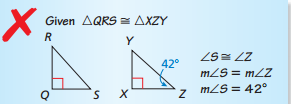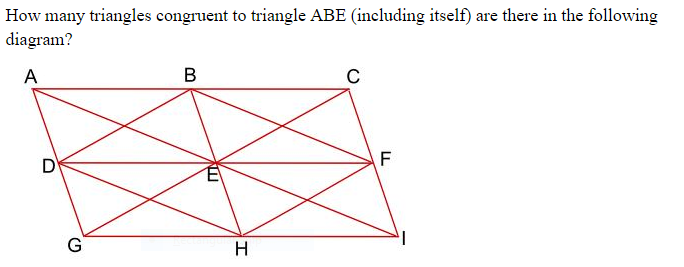3.5 In addition to Score 3.0 performance, the student demonstrates in-depth inferences and applications regarding the more complex content with partial success. Proficient “The Standard.” The student demonstrates no major errors or omissions regarding any of the information and processes that were end of instruction expectations.    Use congruence to solve problems.    Use congruence to show relationships in geometric figures (using CPCTC) Two door stops have cross sections that are right triangles.  They both have a 200 angle and the length of the side between the 900 and the 200 angles are equal.  Are the cross sections congruent?  Explain.   Solve for x and y: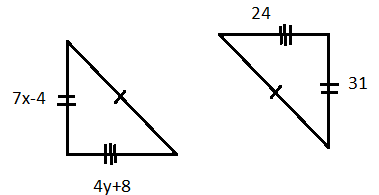2.5 The student demonstrates no major errors or omissions regarding the simpler details and processes (Score 2.0 content) and partial knowledge of the more complex ideas and processes (Score 3.0 content). Progressing The student demonstrates no major errors or omissions regarding the simpler details and processes but exhibits major errors or omissions regarding the more complex ideas and processes (Score 3.0 content). recognizes the congruence criteria for triangles (SAS, ASA, etc.) recognize and use specific terminology such as: Corresponding angles, corresponding sides, congruence statement Identity all the pairs of corresponding sides and angles. Then write a congruence statement.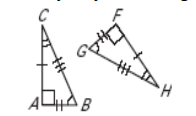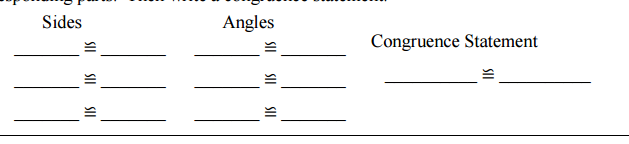1.5 The student demonstrates partial knowledge of the simpler details and processes (Score 2.0 content) but exhibits major errors or omissions regarding the more complex ideas and procedures (Score 3.0 content). Beginning With help, the student demonstrates a partial understanding of some of the simpler details and processes (Score 2.0 content) and some of the more complex ideas and processes (Score 3.0 content). - 0.5 With help, the student demonstrates a partial understanding of some of the simpler details and processes (Score 2.0 content) but not the more complex ideas and processes (Score 3.0 content). 0.0 Even with help, the student demonstrates no understanding or skill. -

## Resources

 Web Vocab

#### MAT-HS.G-SRT.05.b

 MAT-HS Targeted Standards(G) Concept: Geometry(SRT) Domain: Similarity, Right Triangle, and TrigonometryCluster: Prove theorems involving similarity MAT-HS.G-SRT.05.b Use similarity criteria to solve problems in geometric figures.

• I can
• I can

• I can
• I can

• I can
• I can

• I can
• I can

## Proficiency Scale

 Score Description Sample Activity Advanced In addition to Score 3.0, the student demonstrates in-depth inferences and applications regarding more complex material that go beyond end of instruction expectations.3.5 In addition to Score 3.0 performance, the student demonstrates in-depth inferences and applications regarding the more complex content with partial success. Proficient “The Standard.” The student demonstrates no major errors or omissions regarding any of the information and processes that were end of instruction expectations. use similarity properties and proportions to solve for missing measurements use similarity to prove relationships in geometric figures Find the height of the tree.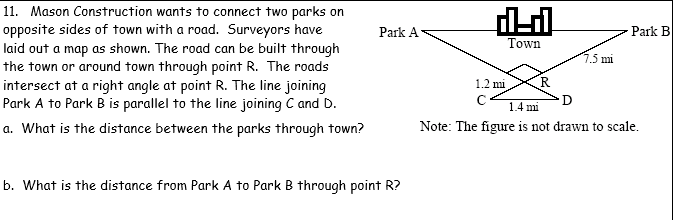2.5 The student demonstrates no major errors or omissions regarding the simpler details and processes (Score 2.0 content) and partial knowledge of the more complex ideas and processes (Score 3.0 content). Progressing The student demonstrates no major errors or omissions regarding the simpler details and processes but exhibits major errors or omissions regarding the more complex ideas and processes (Score 3.0 content). can solve provided proportionality problems can recognize vocabulary such as congruence, similarity, scale factor, ratio and proportion recognizes the similarity criteria for triangles (SAS, SSS, AA) Identify if the given triangles are similar. If they are similar, give the scale factor.Solve for x.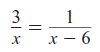1.5 The student demonstrates partial knowledge of the simpler details and processes (Score 2.0 content) but exhibits major errors or omissions regarding the more complex ideas and procedures (Score 3.0 content). Beginning With help, the student demonstrates a partial understanding of some of the simpler details and processes (Score 2.0 content) and some of the more complex ideas and processes (Score 3.0 content). - 0.5 With help, the student demonstrates a partial understanding of some of the simpler details and processes (Score 2.0 content) but not the more complex ideas and processes (Score 3.0 content). 0.0 Even with help, the student demonstrates no understanding or skill. -

## Resources

 Web Vocab

#### MAT-HS.G-SRT.05.c

 MAT-HS Targeted Standards(G) Concept: Geometry(SRT) Domain: Similarity, Right Triangle, and TrigonometryCluster: Prove theorems involving similarity MAT-HS.G-SRT.05.c Use similarity criteria to prove relationships in geometric figures.

• I can
• I can

• I can
• I can

• I can
• I can

• I can
• I can

## Proficiency Scale

 Score Description Sample Activity Advanced In addition to Score 3.0, the student demonstrates in-depth inferences and applications regarding more complex material that go beyond end of instruction expectations.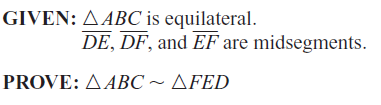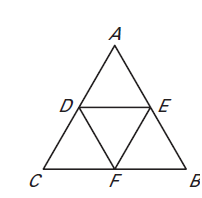3.5 In addition to Score 3.0 performance, the student demonstrates in-depth inferences and applications regarding the more complex content with partial success. Proficient “The Standard.” The student demonstrates no major errors or omissions regarding any of the information and processes that were end of instruction expectations. Use AA, SSS, and SAS Similarity Theorems to prove triangles similar Justify conclusions using valid mathematical arguments. Sketch the triangles using the given description. Explain/Justify whether the two triangles can be similar.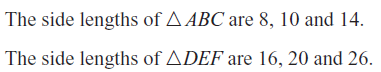2.5 The student demonstrates no major errors or omissions regarding the simpler details and processes (Score 2.0 content) and partial knowledge of the more complex ideas and processes (Score 3.0 content). Progressing The student demonstrates no major errors or omissions regarding the simpler details and processes but exhibits major errors or omissions regarding the more complex ideas and processes (Score 3.0 content). recognize vocabulary such as similarity, corresponding sides, and proportional recognizes the similarity criteria for triangles (SAS, AA, SSS) Are the triangles similar? If so, state the similarity and the postulate or theorem that justifies your answer.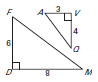1.5 The student demonstrates partial knowledge of the simpler details and processes (Score 2.0 content) but exhibits major errors or omissions regarding the more complex ideas and procedures (Score 3.0 content). Beginning With help, the student demonstrates a partial understanding of some of the simpler details and processes (Score 2.0 content) and some of the more complex ideas and processes (Score 3.0 content). - 0.5 With help, the student demonstrates a partial understanding of some of the simpler details and processes (Score 2.0 content) but not the more complex ideas and processes (Score 3.0 content). 0.0 Even with help, the student demonstrates no understanding or skill. -

## Resources

 Web Vocab

#### MAT-HS.G-SRT.06

 MAT-HS Targeted Standards(G) Concept: Geometry(SRT) Domain: Similarity, Right Triangle, and TrigonometryCluster: Define trigonometric ratios and solve problems involving right triangles. MAT-HS.G-SRT.06 Understand that by similarity, side ratios in right triangles are properties of the angles in the triangle, leading to definitions of trigonometric ratios for acute angles.

## Student Learning Targets:

### Knowledge Targets

• I can show that the side ratios are the same in similar right triangles, which leads to the definition of trigonometric ratios for acute angles.

• I can
• I can

• I can
• I can

• I can
• I can

## Proficiency Scale

 Score Description Sample Activity 4.0 In addition to Score 3.0, the student demonstrates in-depth inferences and applications regarding more complex material that go beyond end of instruction expectations. - 3.5 In addition to Score 3.0 performance, the student demonstrates in-depth inferences and applications regarding the more complex content with partial success. 3.0 “The Standard.” The student demonstrates no major errors or omissions regarding any of the information and processes that were end of instruction expectations. - 2.5 The student demonstrates no major errors or omissions regarding the simpler details and processes (Score 2.0 content) and partial knowledge of the more complex ideas and processes (Score 3.0 content). 2.0 The student demonstrates no major errors or omissions regarding the simpler details and processes but exhibits major errors or omissions regarding the more complex ideas and processes (Score 3.0 content). - 1.5 The student demonstrates partial knowledge of the simpler details and processes (Score 2.0 content) but exhibits major errors or omissions regarding the more complex ideas and procedures (Score 3.0 content). 1.0 With help, the student demonstrates a partial understanding of some of the simpler details and processes (Score 2.0 content) and some of the more complex ideas and processes (Score 3.0 content). - 0.5 With help, the student demonstrates a partial understanding of some of the simpler details and processes (Score 2.0 content) but not the more complex ideas and processes (Score 3.0 content). 0.0 Even with help, the student demonstrates no understanding or skill. -

## Resources

 Web Vocab

#### MAT-HS.G-SRT.07

 MAT-HS Targeted Standards(G) Concept: Geometry(SRT) Domain: Similarity, Right Triangle, and TrigonometryCluster: Define trigonometric ratios and solve problems involving right triangles. MAT-HS.G-SRT.07 Explain and use the relationship between the sine and cosine of complementary angles.

## Student Learning Targets:

### Knowledge Targets

• I can show that the side ratios are the same in similar right triangles, which leads to the definition of trigonometric ratios for acute angles.

### Reasoning Targets

• I can explain the relationship between the sine and cosine of complementary angles.

### Skills (Performance) Targets

• I can use the relationship between the sine and cosine of complementary angles.

• I can
• I can

## Proficiency Scale

 Score Description Sample Activity 4.0 In addition to Score 3.0, the student demonstrates in-depth inferences and applications regarding more complex material that go beyond end of instruction expectations. - 3.5 In addition to Score 3.0 performance, the student demonstrates in-depth inferences and applications regarding the more complex content with partial success. 3.0 “The Standard.” The student demonstrates no major errors or omissions regarding any of the information and processes that were end of instruction expectations. - 2.5 The student demonstrates no major errors or omissions regarding the simpler details and processes (Score 2.0 content) and partial knowledge of the more complex ideas and processes (Score 3.0 content). 2.0 The student demonstrates no major errors or omissions regarding the simpler details and processes but exhibits major errors or omissions regarding the more complex ideas and processes (Score 3.0 content). - 1.5 The student demonstrates partial knowledge of the simpler details and processes (Score 2.0 content) but exhibits major errors or omissions regarding the more complex ideas and procedures (Score 3.0 content). 1.0 With help, the student demonstrates a partial understanding of some of the simpler details and processes (Score 2.0 content) and some of the more complex ideas and processes (Score 3.0 content). - 0.5 With help, the student demonstrates a partial understanding of some of the simpler details and processes (Score 2.0 content) but not the more complex ideas and processes (Score 3.0 content). 0.0 Even with help, the student demonstrates no understanding or skill. -

## Resources

 Web Vocab

#### MAT-HS.G-SRT.08

 MAT-HS Targeted Standards(G) Concept: Geometry(SRT) Domain: Similarity, Right Triangle, and TrigonometryCluster: Define trigonometric ratios and solve problems involving right triangles MAT-HS.G-SRT.08 Use trigonometric ratios and the Pythagorean Theorem to solve right triangles in applied problems.

## Student Learning Targets:

### Knowledge Targets

• I can identify the hypotenuse and legs of a right triangle.
• I can choose the correct trigonometric ratio given a right triangle.
• I can identify the appropriate special right triangle.

### Reasoning Targets

• I can identify the hypotenuse and legs of a right triangle.
• I can choose the correct trigonometric ratio given a right triangle.
• I can identify the appropriate special right triangle.

### Skills (Performance) Targets

• I can apply trigonometric ratios and the Pythagorean Theorem to solve real world problems involving right triangles.

• I can
• I can

## Proficiency Scale

 Score Description Sample Activity 4.0 In addition to Score 3.0, the student demonstrates in-depth inferences and applications regarding more complex material that go beyond end of instruction expectations. - 3.5 In addition to Score 3.0 performance, the student demonstrates in-depth inferences and applications regarding the more complex content with partial success. 3.0 “The Standard.” The student demonstrates no major errors or omissions regarding any of the information and processes that were end of instruction expectations. - 2.5 The student demonstrates no major errors or omissions regarding the simpler details and processes (Score 2.0 content) and partial knowledge of the more complex ideas and processes (Score 3.0 content). 2.0 The student demonstrates no major errors or omissions regarding the simpler details and processes but exhibits major errors or omissions regarding the more complex ideas and processes (Score 3.0 content). - 1.5 The student demonstrates partial knowledge of the simpler details and processes (Score 2.0 content) but exhibits major errors or omissions regarding the more complex ideas and procedures (Score 3.0 content). 1.0 With help, the student demonstrates a partial understanding of some of the simpler details and processes (Score 2.0 content) and some of the more complex ideas and processes (Score 3.0 content). - 0.5 With help, the student demonstrates a partial understanding of some of the simpler details and processes (Score 2.0 content) but not the more complex ideas and processes (Score 3.0 content). 0.0 Even with help, the student demonstrates no understanding or skill. -

## Resources

 Web Vocab

#### MAT-HS.G-SRT.08.a

 MAT-HS Targeted Standards(G) Concept: Geometry(SRT) Domain: Similarity, Right Triangle, and TrigonometryCluster: Define trigonometric ratios and solve problems involving right triangles MAT-HS.G-SRT.08.a Use special right triangles (30°-60°-90° and 45°-45°-90°), trigonometric ratios and the Pythagorean Theorem to solve right triangles in applied problems.   (Mastery of the Pythagorean Theorem is an 8th grade standard.)

• I can
• I can

• I can
• I can

• I can
• I can

• I can
• I can

## Proficiency Scale

 Score Description Sample Activity Advanced In addition to Score 3.0, the student demonstrates in-depth inferences and applications regarding more complex material that go beyond end of instruction expectations. The student will be able to solve complex, multi step trigonometry and Pythagorean Theorem problems in applied situations.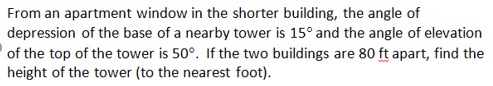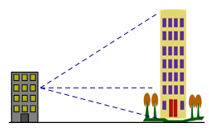3.5 In addition to Score 3.0 performance, the student demonstrates in-depth inferences and applications regarding the more complex content with partial success. Proficient “The Standard.” The student demonstrates no major errors or omissions regarding any of the information and processes that were end of instruction expectations. The student will be able to solve application problems involving trigonometry and Pythagorean Theorem.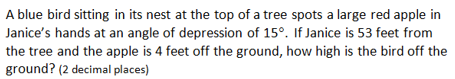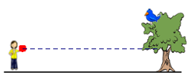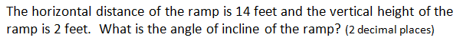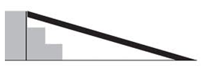The bottom of a ladder must be placed 3 feet from a wall.  The ladder is 12 feet long.  How far above the ground does the ladder touch the wall? 2.5 The student demonstrates no major errors or omissions regarding the simpler details and processes (Score 2.0 content) and partial knowledge of the more complex ideas and processes (Score 3.0 content). Progressing The student demonstrates no major errors or omissions regarding the simpler details and processes but exhibits major errors or omissions regarding the more complex ideas and processes (Score 3.0 content). The student can recognize and recall terminology to answer questions        ·  Angle of elevation        · Angle of depression        · Hypotenuse and legs of a right triangle The student can set up trigonometric ratios and solve a basic trigonometry problem. The student can correctly draw and label a diagram modeling the situation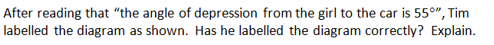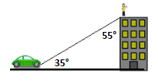Solve for x: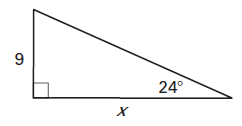1.5 The student demonstrates partial knowledge of the simpler details and processes (Score 2.0 content) but exhibits major errors or omissions regarding the more complex ideas and procedures (Score 3.0 content). Beginning With help, the student demonstrates a partial understanding of some of the simpler details and processes (Score 2.0 content) and some of the more complex ideas and processes (Score 3.0 content). - 0.5 With help, the student demonstrates a partial understanding of some of the simpler details and processes (Score 2.0 content) but not the more complex ideas and processes (Score 3.0 content). 0.0 Even with help, the student demonstrates no understanding or skill. -

## Resources

 Web Vocab

Page: (Previous)   1  2  3  4  5  6  (Next)
ALL